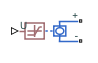# PS Sensor

Generic linear transducer with electrical output

•Libraries:
Simscape / Electrical / Sensors & Transducers

## Description

The PS Sensor block represents a generic linear sensor. The block converts the physical signal input U into an electrical output Y across the + and - ports. The Output type parameter value determines which of the following electrical outputs the block produces:

• Output voltage

• Output current

• Output resistance

Y is related to U as Y = max(min(A · U + B,Ymax),Ymin), where Ymin and Ymax are minimum and maximum limits on the output, respectively.

## Ports

### Input

expand all

Physical input signal.

### Conserving

expand all

Electrical conserving port associated with the PS sensor positive voltage.

Electrical conserving port associated with the PS sensor negative voltage.

## Parameters

expand all

Indicates whether the sensor output is a ```Variable voltage``` of Y V, a ```Variable current``` of Y A, or `Variable resistor` with a value of Y Ω.

Sensitivity of the output Y with respect to the input U, dY/dU.

Output when the input U is zero. The output does not exceed the limits Ymax and Ymin.

Upper limit on the sensor output. The following table shows the units of this parameter, which depend on the selected value of the Output type parameter.

Output typeUnits
`Variable voltage`V
`Variable current`A
`Variable resistor`

Lower limit on the sensor output. The following table shows the units of this parameter, which depend on the selected value of the Output type parameter.

Output typeUnits
`Variable voltage`V
`Variable current`A
`Variable resistor`

If you select `Variable resistance` for the parameter, the minimum resistance Ymin must be greater than zero.

## Version History

Introduced in R2008a